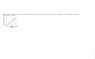# Unraveling the Stress-Strain Curve: Find Young's Modulus & Yield Strength

• rgold

## Homework Statement

The figure shows the stress-strain curve for a material. The scale of the stress axis is set by s = 280, in units of 10^6 N/m2. What are (a) the Young's modulus and (b) the approximate yield strength for this material?

E=stress/strain

## The Attempt at a Solution

so i know that for part a i needa divide 280/.004 but I am not sure what it means to use units of 10^6. also shouldn't part b be 280? the unit that is correct is N/m^2 (its an online grading program that told me this is correct) and i cannot answer a 10^6 or any 10^... (the system won't read it) what am i not understanding?

#### Attachments

•physics.jpg
7.9 KB · Views: 1,443

## The Attempt at a Solution

so i know that for part a i needa divide 280/.004 but I am not sure what it means to use units of 10^6. also shouldn't part b be 280? the unit that is correct is N/m^2 (its an online grading program that told me this is correct) and i cannot answer a 10^6 or any 10^... (the system won't read it) what am i not understanding?

It means that if you enter [answer] this will be interpreted as [answer] x 106 N/m2
e.g. if you put in 6 your answer will be interpreted as 6 Mega Pascals

so shouldn't the answer to part a be .07 n/m^2?

so shouldn't the answer to part a be .07 n/m^2?

What are the units of the '280' ?

What does 280 / 0.004 equal? What are it's units?

280*10^6
so then the answer would be 7e10 but rly 7e4 bc of how the the program will interpret it?

280*10^6
so then the answer would be 7e10 but rly 7e4 bc of how the the program will interpret it?

You still didn't provide any units but yes you are on the right track.

280 MPa / 0.004 = 70 GPa = 70 000 MPa = 70 000 x 106 N / m2

so my final answer would be 70000e6 N/m^2 for part a and 250e6 N/m^2 for part b?